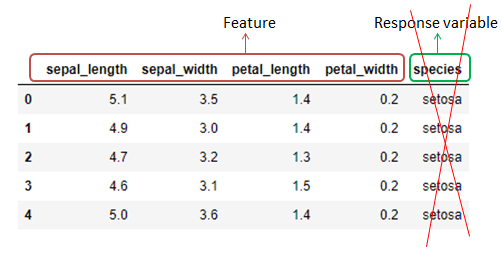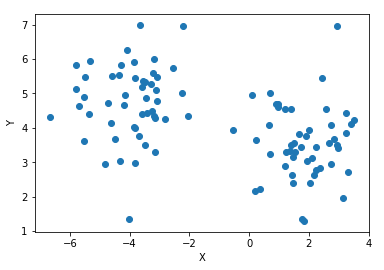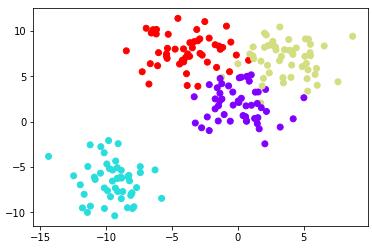# K-Means clustering with Scikit-Learn

K-Means clustering is an unsupervised learning algorithm.

## Features and response variable

To understand unsupervised learning, first of all we will get to know what is a feature and a response variable. Let’s take the example of the famous iris dataset.The columns which represent the features of the iris flower are named as features. (sepal & petal length and width)

The column(s) which represent the type of the flower is the response variable(species). The response variable is the column value which is normally predicted by machine learning implementation.

OK, that is all about features and response variables. Now, coming to unsupervised learning.

## Unsupervised learning

Unsupervised learning, in short means, implementing machine learning on data that has only the features. The important thing to note is, there is no response variable associated with the data.It is like, performing machine learning on the above iris dataset without the response variable species.

An immediate question that arises in our mind here is, what is the use of unsupervised learning if there is nothing to predict??

It is true that we will not be able to predict any response variable out of the process. But we will be able to analyze the behavior and distribution of the features. Also, the relationship between the features can be studied.

And based on that, useful information can be derived out of the data, which is more than the mere prediction of a response variable. This is why K-Means is mostly used as a part of Exploratory data analysis.

Now, its time to discuss the K-Means Clustering algorithm which is one of the Unsupervised Machine learning algorithms.

## K-Means Clustering algorithm

Based on two features X and Y in a scatter plot, we will spread a random set of data points on a scatter plot.K-Means algorithm requires an input called ‘K’ from us. So, what is K?

K denotes the number of clusters to be created out of the available points. Based on our requirement, we can specify the number of clusters/classifications to be created from the data points.

For the above scatter plot, if K= 2, then we can expect the points to be grouped like below.So, based on the K value, we can expect K-Means to classify the data points.

To understand the K-Means algorithm better, we will implement the K-Means on a synthetic set of data created using Scikit-Learn.

## K-Means algorithm implementation

### Importing Necessary libraries

```import matplotlib.pyplot as plt
%matplotlib inline
import seaborn as sns
```

### Synthetic data creation

Instead of working on real-world datasets, we will create our own artificial dataset for K-Means implementation.

```from sklearn.datasets import make_blobs

data = make_blobs(n_samples=200, n_features=2, centers=4,
cluster_std=1.8, random_state=101)
```

Click to know more about the synthetic dataset creation.

### Visualizing the synthetic dataset

Let’s see how our dataset looks like on a scatter plot.

```plt.scatter(data[:,0],data[:,1],c=data,cmap='rainbow')
```There are 4 groups of points in the above scatter plot, which is a direct representation of the centers(4) attribute which we specified in the make_blobs function.

### K-Means implementation

Let’s import K-Means.

```from sklearn.cluster import KMeans
```

Now, we will define and declare K-Means with the number of clusters required.

```kmeans = KMeans(n_clusters= 4)
```

Let’s fit the created data into our K-Means algorithm with the below lines.

```kmeans.fit(data)
```
```KMeans(algorithm='auto', copy_x=True, init='k-means++', max_iter=300,
n_clusters=4, n_init=10, n_jobs=1, precompute_distances='auto',
random_state=None, tol=0.0001, verbose=0)```

### Predicting the clusters of each point

From the scatter plot shown above, it is evident that there are 4 groups of points. And it is understandable that there is a center for each cluster. First, of all, we will try to locate the centers of each point cluster.

```kmeans.cluster_centers_
```
```array([[-9.46941837, -6.56081545],
[ 3.71749226,  7.01388735],
[-4.13591321,  7.95389851],
[-0.0123077 ,  2.13407664]])```

The above array output provides the center coordinates of each cluster.

Now, we will take a look at the predicted cluster category of each data point.

```kmeans.labels_
```
```array([2, 1, 3, 1, 1, 0, 1, 3, 1, 3, 2, 3, 1, 1, 2, 3, 1, 3, 0, 2, 0, 3,
3, 0, 2, 0, 0, 3, 1, 1, 2, 0, 1, 3, 3, 2, 0, 0, 0, 3, 0, 2, 2, 2,
3, 1, 2, 3, 0, 3, 3, 2, 1, 3, 0, 2, 3, 3, 2, 1, 0, 1, 0, 2, 1, 3,
0, 1, 1, 0, 1, 3, 0, 3, 0, 1, 1, 3, 2, 3, 3, 0, 1, 0, 3, 3, 3, 2,
3, 0, 0, 0, 0, 3, 3, 0, 1, 2, 0, 1, 3, 0, 3, 3, 1, 3, 0, 1, 0, 0,
1, 2, 2, 1, 0, 1, 2, 2, 1, 2, 3, 2, 3, 2, 3, 1, 2, 3, 0, 2, 2, 2,
3, 0, 0, 2, 1, 2, 1, 3, 0, 1, 0, 2, 2, 1, 3, 0, 2, 2, 2, 2, 3, 1,
3, 2, 1, 1, 1, 3, 1, 3, 3, 2, 0, 2, 3, 1, 2, 3, 1, 3, 2, 1, 3, 2,
1, 1, 0, 1, 2, 0, 0, 2, 0, 0, 0, 0, 0, 3, 0, 1, 1, 2, 0, 3, 1, 1,
0, 3])```

The above output is hard to visualize, interpret or understand. So we will create a plot that provides the data with original classification and the way in which the K-Means classified our data.

```fig , (ax1, ax2) = plt.subplots(1,2, sharey= True, figsize=(10,6))

ax1.set_title('K Means')
ax1.scatter(data[:,0], data[:,1], c=kmeans.labels_
,cmap='rainbow')

ax2.set_title('Original')
ax2.scatter(data[:,0], data[:,1], c=data,
cmap='rainbow')
```It is immaterial to take into consideration, the differences in colors between the original and the K-Means plot.

## Conclusion

From the above plot, we can interpret that the classification by K-Means is more or less similar to the original. Also, take note that there is a very slight difference between the top two clusters in the above plots. So, what do you infer from the above plot, do comment below.

I also, recommend you to check the below post to know how to create artificial/synthetic datasets in Scikit-learn.

make_blobs: How to create Artificial datasets in Python?